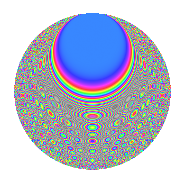# Properties

 Label 804.2.lLevel 804 Weight 2 Character orbit l Rep. character $$\chi_{804}(431,\cdot)$$ Character field $$\Q(\zeta_{6})$$ Dimension 264 Newforms 1 Sturm bound 272 Trace bound 0

# Related objects

## Defining parameters

 Level: $$N$$ = $$804 = 2^{2} \cdot 3 \cdot 67$$ Weight: $$k$$ = $$2$$ Character orbit: $$[\chi]$$ = 804.l (of order $$6$$ and degree $$2$$) Character conductor: $$\operatorname{cond}(\chi)$$ = $$804$$ Character field: $$\Q(\zeta_{6})$$ Newforms: $$1$$ Sturm bound: $$272$$ Trace bound: $$0$$

## Dimensions

The following table gives the dimensions of various subspaces of $$M_{2}(804, [\chi])$$.

Total New Old
Modular forms 280 280 0
Cusp forms 264 264 0
Eisenstein series 16 16 0

## Trace form

 $$264q - 2q^{4} - 3q^{6} - 12q^{9} + O(q^{10})$$ $$264q - 2q^{4} - 3q^{6} - 12q^{9} + 2q^{10} + 2q^{12} - 18q^{16} + q^{18} - 4q^{22} - 248q^{25} + 7q^{30} - 38q^{34} + 13q^{36} + 4q^{37} + 48q^{40} - 12q^{42} + 48q^{45} - 2q^{46} - 11q^{48} + 128q^{49} + 32q^{52} - 8q^{54} - 2q^{57} - 32q^{58} + 68q^{60} - 8q^{61} + 4q^{64} + 30q^{66} - 40q^{69} - 140q^{70} + 108q^{72} - 4q^{73} - 120q^{76} + 44q^{78} - 28q^{81} - 64q^{82} + 33q^{84} + 16q^{85} - 14q^{88} + 42q^{90} + 16q^{93} - 12q^{94} - 4q^{96} + 20q^{97} + O(q^{100})$$

## Decomposition of $$S_{2}^{\mathrm{new}}(804, [\chi])$$ into irreducible Hecke orbits

Label Dim. $$A$$ Field CM Traces $q$-expansion
$$a_2$$ $$a_3$$ $$a_5$$ $$a_7$$
804.2.l.a $$264$$ $$6.420$$ None $$0$$ $$0$$ $$0$$ $$0$$